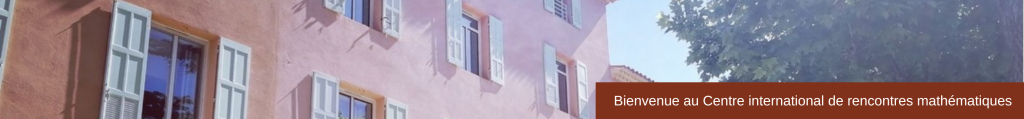## SLIDES

• José M. Arrieta (Complutense University of Madrid)

Thin domains with a locally periodic highly oscillatory boundary   (pdf)

• Mark Ashbaugh (University of Missouri)

A Sharp Lower Bound for the First Eigenvalue of the Vibrating Clamped Plate under Compression   (pdf)

• Catherine Bandle (University of Basel) and Alfred Wagner ( RWTH Aachen)

On an eigenvalue problem with infinitely many positive and negative eigenvalues   (pdf)

• Rafael Benguria ( P. U. Catolica de Chile)

The Brezis-Nirenberg Problem for the Laplacian with a singular drift in Rn, Sn and Hn   (pdf)

• Chiara Bianchini (University of Florence)

Wulff Shape characterization in overdetermined anisotropic elliptic problems   (pdf)

• Virginie Bonnaillie-Noel (ENS Paris)

Spectral minimal partitions for a family of tori   (pdf)

• Friedemann Brock (University of Rostock)

Some isoperimetric inequalities on RN with respect to weights x alpha   (pdf)

• Bruno Colbois (Université de Neuchâtel)

Bounds for the spectrum of the magnetic Laplacian   (pdf)

• Gisella Croce (Université Le Havre Normandie)

On the selection of solutions to a nonlinear PDE system   (pdf)

• Gianni Dal Maso (SISSA, Trieste)

Existence and uniqueness of dynamic evolutions for a peeling test in dimension one   (pdf)

• Marc Dambrine (Université de Pau)

Taking uncertainties into account in numerical shape optimization   (pdf)

• Ilaria Fragalà (Politecnico di Milano)

Some new inequalities for the Cheeger constant   (pdf)

• Nicola Fusco (University of Naples Federico II)

A stability result for the first eigenvalue of the p-Laplacian   (pdf)

• Alessandro Giacomini (University of Brescia)

Shape optimization with Robin conditions and free discontinuity problems   (pdf)

• Alexandre Girouard ( Université de Laval)

The Steklov spectrum and coarse discretization   (pdf)

• Katie Gittins (Université de Neuchâtel)

Asymptotic optimal sets for the eigenvalues of the Laplacian   (pdf)

• Bernd Kawohl (University of Köln)

Two dimensions are easier   (pdf)

• Jimmy Lamboley (Université Paris-Dauphine)

Regularity for functionals involving perimeter   (pdf)

• Richard Laugesen (University of Illinois)

Optimal stretching for lattice points and eigenvalues   (pdf)

• Dario Mazzoleni ( University of Turin)

Regularity of the optimal sets for spectral functionals: Part II, some generalizations   (pdf)

• Nikolai Nadirashvili ( Aix-Marseille Université)

Isoperimetric inequalities for spectrum of Laplacian on surfaces   (pdf)

• Carlo Nitsch (University of Naples Federico II)

Symmetry breaking for a problem in optimal insulation   (pdf)

• Yannick Privat (Université Pierre et Marie Curie)

Geometrical properties of resources optimal arrangements for species survival   (pdf)

• Paolo Salani (University of Florence)

About the stability of Borell-Brascamp-Lieb inequalities   (pdf)

• Kathrin Stollenwerk (RWTH Aachen University)

Optimal shape of a domain which minimizes the buckling load of a clamped plate   (pdf)

• Susanna Terracini (University of Turin)

Regularity of the optimal sets for spectral functionals – Part I: sum of eigenvalues   (pdf)

• Cristina Trombetti (University of Naples Federico II)

On the Stability of the Bossel-Daners Inequality   (pdf)